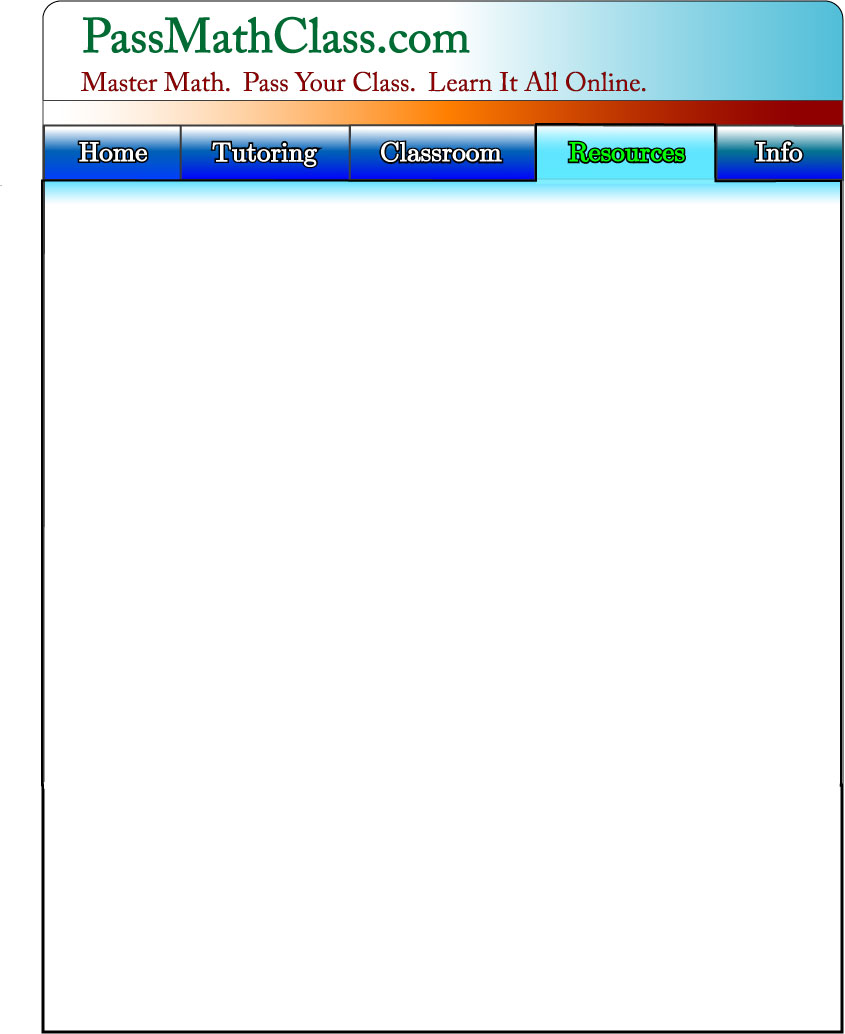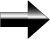A Table Of Important Symbols

This is a list of the important symbols you will use in almost every math class.  Try to learn as many of these symbols as you can.

Main Pre-Algebra Page=       is equal to

≠       is not equal to

≈       is approximately equal to

±       plus or minus

≤ x     is less than or equal to x.

≥ x     is greater than or equal to x

∞      infinity

∑      The Summation Symbol

π       Pi ≈ 3.14159265359